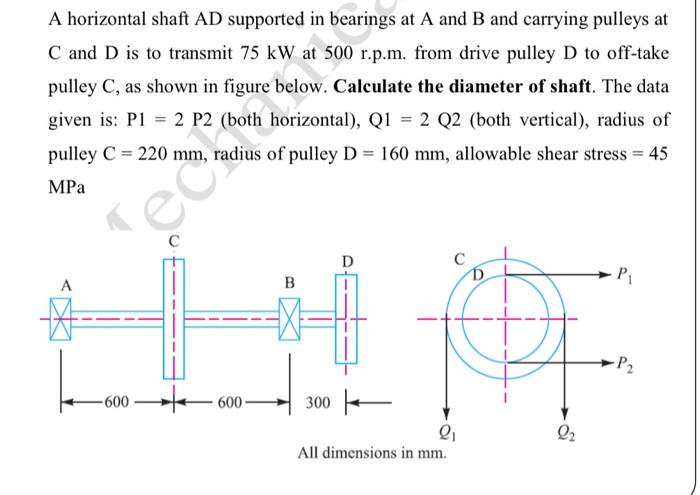# Question A horizontal shaft AD supported in bearings at A and B and carrying pulleys at C and D is to transmit 75 kW at 500 r.p.m. from drive pulley D to off-take pulley C, as shown in figure below. Calculate the diameter of shaft. The data given is: P1 = 2 P2 (both horizontal), Q1 = 2 Q2 (both vertical), radius of pulley C = 220 mm, radius of pulley D = 160 mm, allowable shear stress = 45 MPа BP -- - -- 600 +-600— 300 | All dimensions in mm.OCJO8I The Asker · Mechanical EngineeringTranscribed Image Text: A horizontal shaft AD supported in bearings at A and B and carrying pulleys at C and D is to transmit 75 kW at 500 r.p.m. from drive pulley D to off-take pulley C, as shown in figure below. Calculate the diameter of shaft. The data given is: P1 = 2 P2 (both horizontal), Q1 = 2 Q2 (both vertical), radius of pulley C = 220 mm, radius of pulley D = 160 mm, allowable shear stress = 45 MPа BP -- - -- 600 +-600— 300 | All dimensions in mm.
More
Transcribed Image Text: A horizontal shaft AD supported in bearings at A and B and carrying pulleys at C and D is to transmit 75 kW at 500 r.p.m. from drive pulley D to off-take pulley C, as shown in figure below. Calculate the diameter of shaft. The data given is: P1 = 2 P2 (both horizontal), Q1 = 2 Q2 (both vertical), radius of pulley C = 220 mm, radius of pulley D = 160 mm, allowable shear stress = 45 MPа BP -- - -- 600 +-600— 300 | All dimensions in mm.